GATE  >  Test: Representation of Signed Numbers

# Test: Representation of Signed Numbers

Test Description

## 10 Questions MCQ Test Digital Circuits | Test: Representation of Signed Numbers

Test: Representation of Signed Numbers for GATE 2023 is part of Digital Circuits preparation. The Test: Representation of Signed Numbers questions and answers have been prepared according to the GATE exam syllabus.The Test: Representation of Signed Numbers MCQs are made for GATE 2023 Exam. Find important definitions, questions, notes, meanings, examples, exercises, MCQs and online tests for Test: Representation of Signed Numbers below.
Solutions of Test: Representation of Signed Numbers questions in English are available as part of our Digital Circuits for GATE & Test: Representation of Signed Numbers solutions in Hindi for Digital Circuits course. Download more important topics, notes, lectures and mock test series for GATE Exam by signing up for free. Attempt Test: Representation of Signed Numbers | 10 questions in 30 minutes | Mock test for GATE preparation | Free important questions MCQ to study Digital Circuits for GATE Exam | Download free PDF with solutions
 1 Crore+ students have signed up on EduRev. Have you?
Test: Representation of Signed Numbers - Question 1

### Which of the following is the smallest 4-bit negative number stored in its 2's complement representation?

Detailed Solution for Test: Representation of Signed Numbers - Question 1

The smallest negative number is the largest binary value.
1111 is -1, 1110 is -2, 1101 is -3, etc down to 1000 which represents -8.

Test: Representation of Signed Numbers - Question 2

### The I's complement of the binary number (101100)2 is:

Detailed Solution for Test: Representation of Signed Numbers - Question 2

Concept:
1's complement of Binary: 1's complement of a Binary number is defined by the value obtained by inverting all the bit, i.e, 0 as 1 and 1 as 0.

Calculation:
The 1's complement of the given binary digit will be:
(101100) → (010011)

Test: Representation of Signed Numbers - Question 3

### Perform the subtraction and represent your answer in 2’s complement form (10010)2 - (10111)2

Detailed Solution for Test: Representation of Signed Numbers - Question 3

Concept:
If number is positive; MSB = 0
Then 2’s complement will be the same
If number is negative; MSB = 1
Then 2’s complement will be different from its obtained result

Calculation:
Given,
(10010)2 - (10111)2
(10010)2 + 2’s complement of (10111)2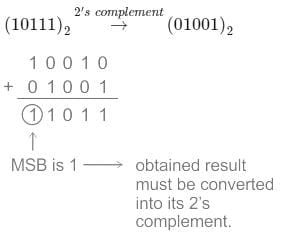i.e. (11011)2 = -[2’s complement of 11011]
= -
∴ (10010)2 - (10111)2 = -(00101)2

Test: Representation of Signed Numbers - Question 4

The difference in the dynamic range of 32-bit binary number(B) and floating-point number(F) is?
Standard Format for B and F as given below :
B=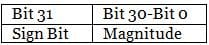F=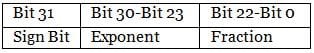Detailed Solution for Test: Representation of Signed Numbers - Question 4

Concept:
The dynamic range in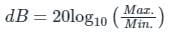In fixed point, unsigned integer representation using N-bit, the range of Max to Min is 2N to 1
In fixed-point signed integer representation using N-bit, the range of Max to Min number is 2N-1 to 1
Calculation:
The dynamic range of a 32-bit binary number B is: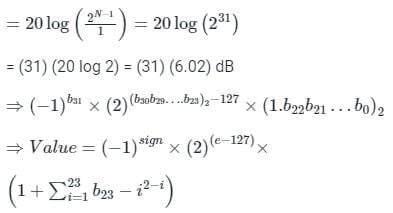Exponent = e – 127 varies from –127 to 128,
e = 0 = all bits (b30 ---- b23) are zero.
Now, the dynamic range is determined by the size of the exponent, which is
1 × 2-127(min) to 2128 (max).
Dynamic range: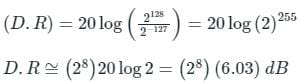Difference in Dynamic Ranges = 6.03 (28 – 31)
So, Option (2) is correct.

*Answer can only contain numeric values
Test: Representation of Signed Numbers - Question 5

No of bits required to represent -6410 in 2’s complement form:

Detailed Solution for Test: Representation of Signed Numbers - Question 5

64 in binary form is represented as:
6410 = (1000000)2
Taking the 1's complement of the above, we get 0111111
Adding 1 to the 1's complement, we get the 2's complement representation of the number, i.e. 1000000.
Since there is a 1 in the LSB, the number is a negative number with value 64.
∴ The 2's complement of -6410 contains 7 bits.

Test: Representation of Signed Numbers - Question 6

The number of 1’s in the 8-bit unsigned representation of 127 in its 2’s complement form is m and that in 1’s complement form is n. What is the value of m : n?

Detailed Solution for Test: Representation of Signed Numbers - Question 6

Concept:
1’s complement representation of a binary number is obtained by toggling all the bits, i.e. replacing 1 with 0, and 0 with 1.
2’s complement representation of a binary number is obtained by adding 1 to the 1’s complement representation.

Application:
(127)10 = (01111111)2
1’s complement representation will be:
1’s complement = 10000000
Number of 1’s is the 1’s complement is, n = 1
Now, the two (2’s) complement representation will be:
2’s complement = 10000000 + 1
= 10000001
Number of 1’s in 2’s complement is, m = 2
∴ The required ratio is m : n = 2 : 1

Test: Representation of Signed Numbers - Question 7

In sign-magnitude numbers

Detailed Solution for Test: Representation of Signed Numbers - Question 7

Signed magnitude representation uses the most significant bit (MSB) a sign bit.
1) If the sign bit is ‘0’ then the number is positive.
2) If the sign bit is ‘1’ then the number is negative.
The remaining bits represent the magnitude of the binary number.
1000101 represents a negative number as the MSB bit is '1'
0101001 represents a positive number as the MSB bit is '0'

Test: Representation of Signed Numbers - Question 8

A register contains a 2’s complement no 10100. Find the value of a register if it is divided by 2

Detailed Solution for Test: Representation of Signed Numbers - Question 8

Given Number is 10100
The Right shift of the content in register is same as the content divided by 2
Apply Right Shift ⇒ 11010
Operation right shift is equivalent to divided by 2

Test: Representation of Signed Numbers - Question 9

X = 01110 and Y = 11001 are two 5-bit binary numbers represented in two’s complement format. The sum of X and Y represented in two’s complement format using 6 bits is

Detailed Solution for Test: Representation of Signed Numbers - Question 9

To represent a given 5-bit number using 6- bits in a 2's complement representation, we simply copy the MSB bit as it is till we get the required 6 bits, i.e.
X = 01110 = 001110
Y = 11001 =111001
Ignoring the carry, we get the addition of the two in 2's complement number as:
∴ x + y = 000111

Test: Representation of Signed Numbers - Question 10

X = 00110 and Y = 10011 are two binary numbers represented in 2's complement format. The sum of X and Y represented in 2's complement format using 5 bits is _____

Detailed Solution for Test: Representation of Signed Numbers - Question 10

X = 00110
since, the MSB = 0
∴ it is a positive number.
Decimal equivalent: 0 + 1 × 22 + 1 × 21 + 0 × 20 = + 6
Y = 10011
since, the MSB = 1
∴ it is a negative number,
We need to take the 2's complement of Y, that is.
1's complement (Y) + 1
01100 + 1
⇒ 01101
Decimal equivalent → 0 + 1 × 23 + 1 × 22 + 0 + 1 × 20 → -13
The sum of X and Y is
+6 - 13 = - 7
The 2's complement of - 7
→ 1's complement of 7 + 1
→ 1's complement of 00111 + 1
→ 11000 + 1 → 11001

## Digital Circuits

76 docs|43 tests
 Use Code STAYHOME200 and get INR 200 additional OFF Use Coupon Code
Information about Test: Representation of Signed Numbers Page
In this test you can find the Exam questions for Test: Representation of Signed Numbers solved & explained in the simplest way possible. Besides giving Questions and answers for Test: Representation of Signed Numbers, EduRev gives you an ample number of Online tests for practice

76 docs|43 tests